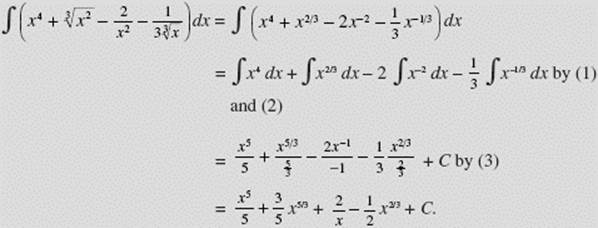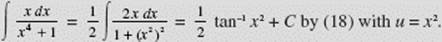﻿ ﻿BASIC FORMULAS - Antidifferentiation - Calculus AB and Calculus BC

## CHAPTER 5 Antidifferentiation

### B. BASIC FORMULAS

Familiarity with the following fundamental integration formulas is essential.All the references in the following set of examples are to the preceding basic formulas. In all of these, whenever u is a function of x, we define du to be u (x) dx; when u is a function of t, we define du to be u (t) dt; and so on.

EXAMPLE 1EXAMPLE 2EXAMPLE 3EXAMPLE 4is integrated most efficiently by using formula (3) with u = 1 − 3x and du = u (x)dx = −3 dx.EXAMPLE 5where u = 2x3 − 1 and du = u (x) dx = 6x2 dx; this, by formula (3), equalsEXAMPLE 6du, where u = 1 − x and du = −1 dx; this, by formula (3) yieldsEXAMPLE 7EXAMPLE 8EXAMPLE 9EXAMPLE 10EXAMPLE 11EXAMPLE 12EXAMPLE 13If the degree of the numerator of a rational function is not less than that of the denominator, divide until a remainder of lower degree is obtained.

EXAMPLE 14EXAMPLE 15EXAMPLE 16with u = 5 + 2 sin x. The absolute-value sign is not necessary here since (5 + 2 sin x) > 0 for all x.

EXAMPLE 17EXAMPLE 18(by long division) = −x − ln |1 − x| + C.

EXAMPLE 19EXAMPLE 20EXAMPLE 21EXAMPLE 22EXAMPLE 23EXAMPLE 24EXAMPLE 25+ C by (3) with u = tan t and du = u (t) dt = sec2 t dt.

EXAMPLE 26EXAMPLE 27by (4)

with u = 1 + 2andEXAMPLE 28with u = cos x;cos 2x + C by (6), where we use the trigonometric identity sin 2x = 2 sin x cos x.

EXAMPLE 29EXAMPLE 30using the trigonometric identityEXAMPLE 31EXAMPLE 32EXAMPLE 33EXAMPLE 34EXAMPLE 35EXAMPLE 36EXAMPLE 37EXAMPLE 38EXAMPLE 39EXAMPLE 40EXAMPLE 41BC ONLY

﻿